Next: About this document ... Up: lab_template Previous: lab_template

Subsections

# Numerical Integration

## Purpose

The purpose of this lab is to give you some experience with using the trapezoidal rule and Simpson's rule to approximate integrals.

## Background

The trapezoidal rule and Simpson's rule are used for approximating area under a curve or the definite integralBoth methods start by dividing the interval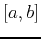into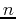subintervals of equal length by choosing a partitionsatisfying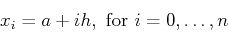where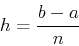is the length of each subinterval. For the trapezoidal rule, the integral over each subinterval is approximated by the area of a trapezoid. This gives the following approximation to the integralFor Simpson's rule, the function is approximated by a parabola over pairs of subintervals. When the areas under the parabolas are computed and summed up, the result is the following approximation.## Maple commands

The commands for the trapezoidal rule and Simpson's rule are in the student package.
>with(student);

The following example will use the function
>f:=x->x^2*exp(x);

This computes the integral of the function from 0 to 2.
>int(f(x),x=0..2);

Using the evalf command provides a decimal approximation.
>evalf(int(f(x),x=0..2));

The command for using the trapezoidal rule is trapezoid. The syntax is very similar to that of the int command. the last argument specifies the number of subintervals to use. In the command below, the number of subintervals is set to 10, but you should experiment with increasing or decreasing this number. Note that Maple writes out the sum and doesn't evaluate it to a number.
>trapezoid(f(x),x=0..2,10);

Putting an evalf command on the outside computes the trapezoidal approximation.
>evalf(trapezoid(f(x),x=0..2,10));

The command for Simpson's rule is very similar.
>simpson(f(x),x=0..2,10);
>evalf(simpson(f(x),x=0..2,10));


## Error term

There is an error term associated with the trapezoidal rule that can be u sed to estimate the error. More precisely, we have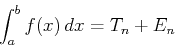where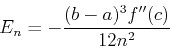for some value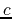between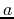and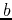that maximizes the second derivative in absolute value. Solving the error formula forguarantees a number of subintervals such that the error term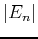is less than some desired tolerance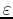. This gives:The way to think about this result is that it gives a value forwhich guarantees that the error of the trapezoidal rule is less than the tolerance. It is generally a very conservative result.

Similarly, the number of subintervals for the simpson rule approximation to guarantee an error smaller thanis## Exercises

1. For the function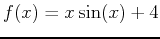, use the trapezoidal rule formula to approximate the area under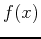over the interval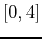ising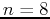. Verify your answer using the trapezoid command in Maple. Repeat this exercise with simpson's rule and Maple's simpson command.

2. For the functionover the interval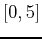, complete the following steps.
(i)
By using Maple's int and possibly evalf commands, find a good approximation to the integral of the function over the given interval.
(ii)
Find the minimum number of subintervalsnecessary to approximate the value of the definite integral with error no greater than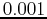. Then do the same with Simpson's rule. Which is more accurate and why?
(iii)
Use the error estimate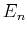for the trapezoidal rule to find a value for, the number of subintervals, that ensuresis withinof the answer in part (i). How close is this answer to the number of subintervals you found in part (ii)?
(Hint: The part of the error term that is most difficult is. To find the maximum value of the second derivative ofover the interval, an approximation based on the plot should suffice. To plot the second derivative ofover the given interval, type the following command in Maple:
plot(abs(diff(f(x),x,x)),x=0..5);

Then, using your mouse, click on the part of the plot that appears to be the maximum. When you click on a plot,coordinates will appear in the upper left hand corner of your Maple window and the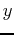coordinate is used for the max.)
(a)
(iv)] Repeat part (iii) using Simpson's rule. (Hint: To maximize the fourth derivative ofover the given interval, type the following command in Maple:
plot(abs(diff(f(x),x,x,x,x)),x=0..5);Next: About this document ... Up: lab_template Previous: lab_template
Dina J. Solitro-Rassias
2013-10-07